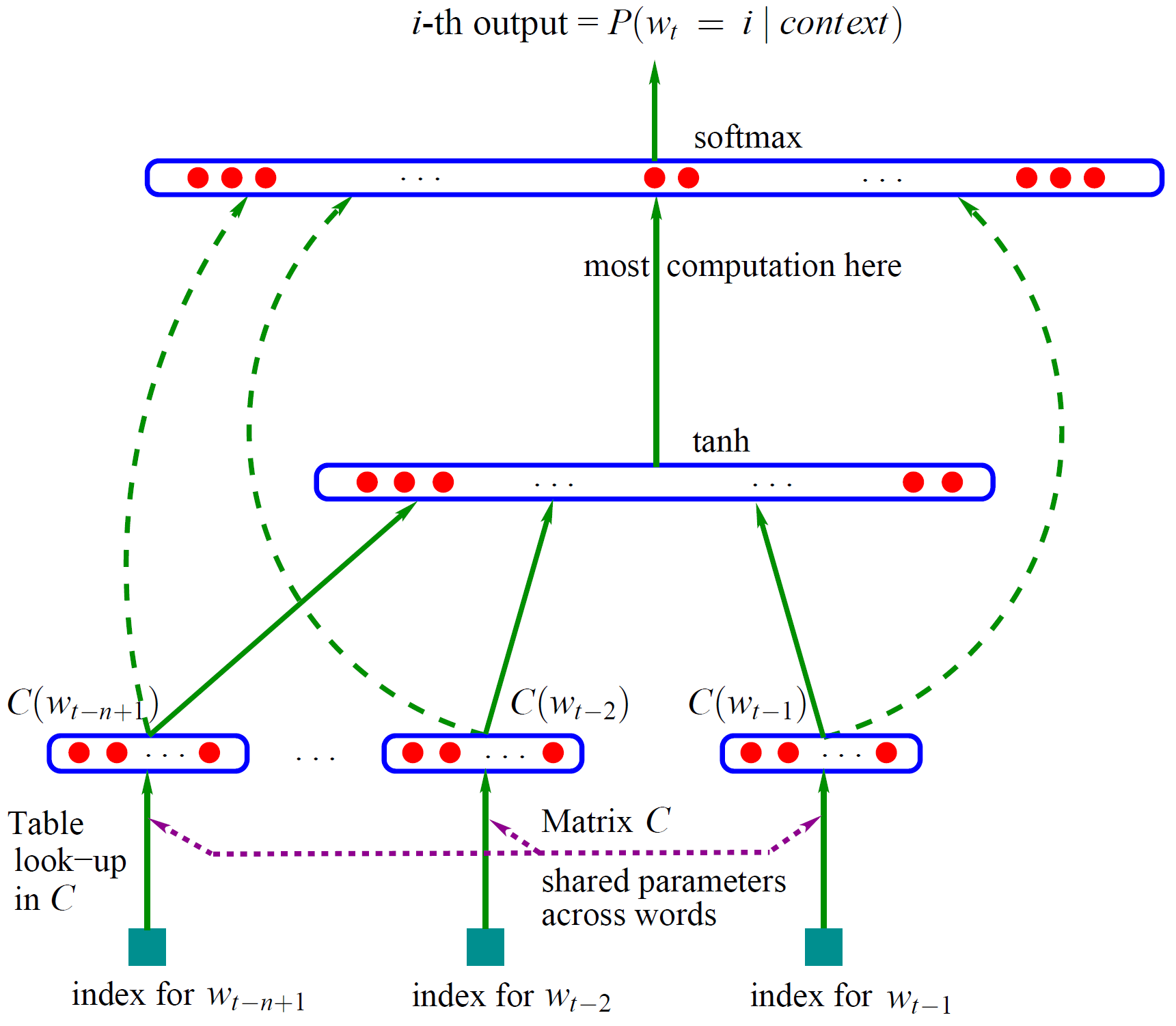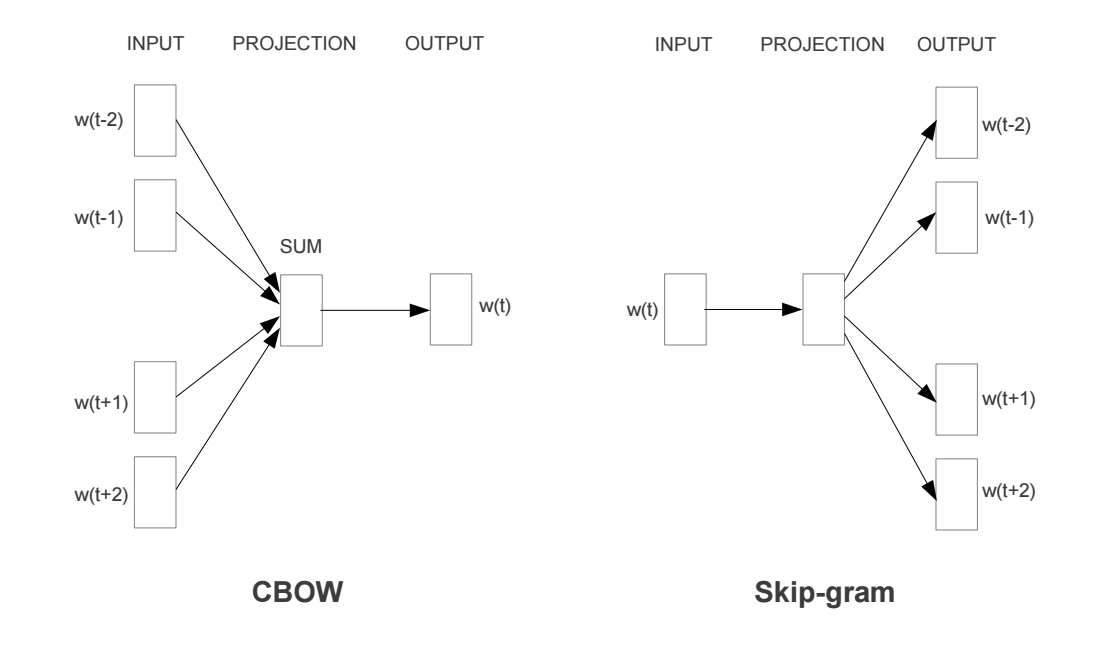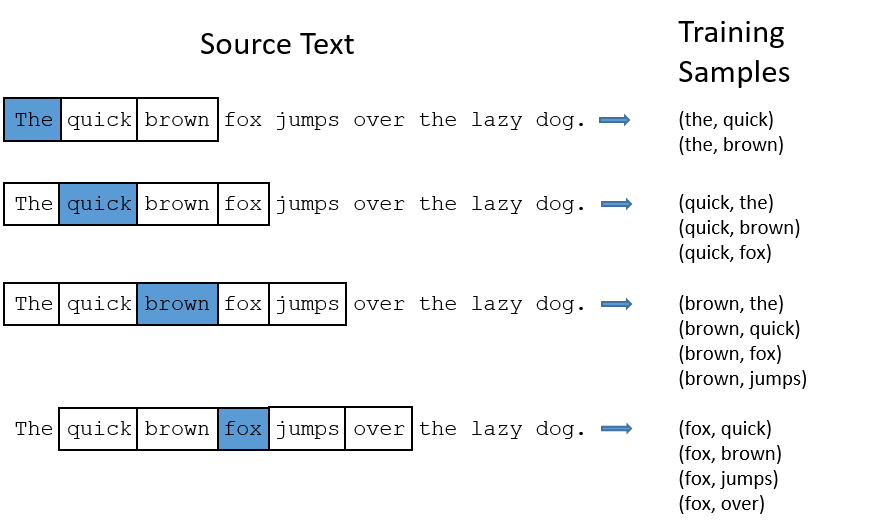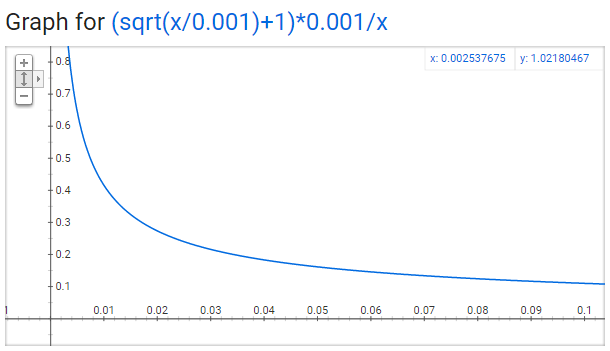# 语言模型

$$P(w_1,w_2,w_3,….,w_n) = P(w_1)\cdot P(w_2 | w_1) \cdot P(w_3 |w_1, w_2)\cdot \ … \ P(w_n|w_1,w_2,…w_{n-1})$$

2003年，Bengio发表了神经网络语言模型(NNLM)论文，其网络结构如下图所示：NNLM包含输入层，隐层，输出层三层神经网络，首先来看输入层，将单词$w_t$的上文(前n个单词)使用one-hot形式表示，并乘以矩阵$C$(跟据one-hot形式可发现是查表操作)。然后将得到的向量拼接在一起得到 $[C(w_{t-n+1}), …C(W_{t-2}), C(W_{t-1})]$ ，其作为隐层的输入。隐层的激活函数为tanh。最后输出层使用Softmax进行词汇表 $V$ 上的分类。矩阵$C$初始是随机初始化的，通过NNLM任务发现，学习后的矩阵$C$是一个密集且包含语义的词向量矩阵。通过one-hot进行相乘可得到每个单词对应的词向量。

one-hot向量长度为词汇表$V$维向量，嵌入维度m可自行设定。在cs224n课程中，矩阵$C$及隐层权重$W$都用来表示词嵌入，后者被成为上下文向量。 在吴恩达课程中，嵌入矩阵即为矩阵$C$。

# Word2vec

Word Embedding的常用技术：word2vec主要任务目标就是为了训练得到词向量，而不再是NNLM的任务。word2vec包含两个模型CBOWSkip-gram。word2vec是由两篇论文完成的，第一篇论文提出了两个模型，第二篇论文提出了两个训练技巧，使训练更加有效可行。

## 模型细节CBOW模型是将中心词的上下文作为输入来进行预测，而Skip-gram是根据中心词来预测其上下文单词。二者其实差不多。现在以Skip-gram为例来介绍下细节。## 隐层细节## 输出层细节

Softmax细节不再阐述，其能将输出进行概率归一化。## 问题

• 将常用词对或短语视为模型中的单个”word”。
• 对频繁的词进行子采样以减少训练样例的数量。
• 在损失函数中使用”负采样(Negative Sampling)”的技术，使每个训练样本仅更新模型权重的一小部分。

## 对频繁词子采样• 对于单词对(‘fox’,’the’)，其对单词’fox’的语义表达并没有什么有效帮助，’the’在每个单词的上下文中出现都非常频繁。
• 预料中有很多单词对(‘the’,…)，我们应更好的学习单词’the’

Word2vec使用子采样技术来解决以上问题，根据单词的频次来削减该单词的采样率。以window size为10为例子，我们删除’the’：

• 当我们训练其余单词时候，’the’不会出现在他们的上下文中。
• 当中心词为’the’时，训练样本数量少于10。

$$P(w_i)=(\sqrt{\frac{z(w_i)}{0.001}} +1) \cdot \frac{0.001}{z(w_i)}$$• $P(w_i) = 1.0$(100%保留)，对应的 $z(w_i)<=0.0026$。(表示词频大于0.0026的单词才会进行子采样)
• $P(w_i) = 0.5$(50%保留)，对应的 $z(w_i)=0.00746$。
• $P(w_i) = 0.033$(3.3%保留)，对应的 $z(w_i)=1.0$。(不可能)

## 负采样(Negative Sampling)

$$P(w_i) = \frac{f(w_i)^{3/4}}{\sum_{j=0}^n(f(w_j)^{3/4})}$$

# Skip-gram 代码实现

Skip-gram的实现有多种方式，比如很多软件包(gsim)已经收录了，可以直接调用。Skip-gram模型并不复杂，网络仅有一个隐层，使用Keras实现也非常简单，但是自己手写实现更更好理解一些。这里讲下自己的实现。主要参考Tensorflow word2vec模型,该实现封装数据集及推理(analogy)等内容，但对神经网络的定义、负采样、NCE损失函数等内容的，虽然并不都是完全底层实现，但对深入理解还是很有帮助的。数据集采用的是20newsgroups，这个数据集有个博士处理后的版本(去除了停用词the等)。20newsgroups数据还是比较粗糙的，整个实现比较随意，源jupyter及训练数据也就没有上传github,但对模型理解还是有帮助的。